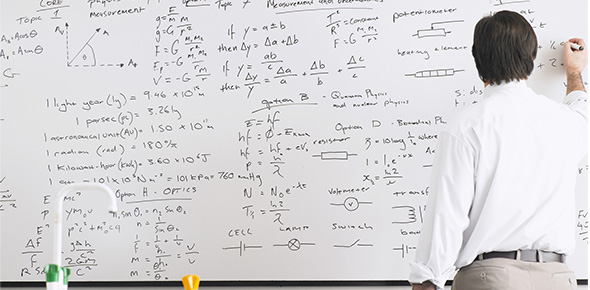# Review For Quiz Alg 2h 2.1 - 2.3

9 Questions | Total Attempts: 48SettingsEquations, Inequalities, Expressions

• 1.
Which of the following is equivalent to the expression below?4x(x - 2) + (x + 8)(x - 3)?A. 5x2- 3x - 24B. 4x2 - 3x - 24C. 5x2 + 13x - 24D. x2 + 9x - 3E. none of the above
• A.

A

• B.

B

• C.

C

• D.

D

• E.

E

• 2.
Evaluate:Can't type square root so sqrt means that.
• 3.
Evaluate:
• 4.
Solve:6 - 4(x + 2) = 6(x - 5)Give answer as decimal or improper fraction
• 5.
Jennifer has a collection of 30 stamps. Some of them are worth \$1.25 each and some of them are worth \$0.75 each. Her collection is worth \$32.50. How many stamps does she have that are worth \$1.25?
• 6.
Evaluate for (x, y) = (-4, 6).
• 7.
Two bicyclists are traveling towards each other. They start 70 miles apart at the same time. One biker is traveling 20 mph and the other biker is traveling 15 mph. How many miles will the faster biker have traveled when they meet?
• 8.
Solve the inequality below:4 < 2x - 8 < 10
• A.

X > 9 or x < 6

• B.

1 < x < 6

• C.

6 < x < 9

• D.

12 < x < 18

• E.

-2 < x < 1

• 9.
Solve for x:
• A.

A.

• B.

B.

• C.

C.

• D.

D.

• E.

E.

Related TopicsBack to top